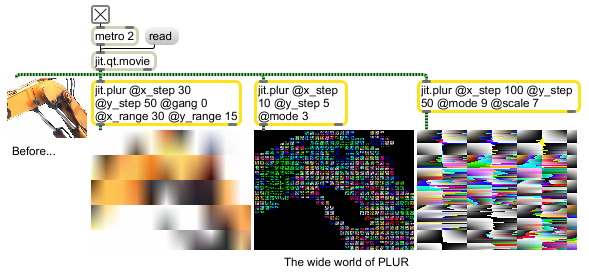# jit.plur

Peace Love Unity Rave

## Description

In its default mode of operation, the jit.plur object performs linear interpolation on incoming matrix frames -- it resamples the image, and then interpolates back to the original size. In its other modes, the object performs a wide variety of variations on theme.

The resampling process uses two different attributes along each axis of the matrix frame. The _step attributes determine how the frame is divided at output. For instance, with x_step set to 5, there will be a resampled rectangle every 5 cells along the horizontal axis. The _range attributes determine how that resampled rectangle is interpolated. If x_range is less than or greater than x_step, different corner points are used for the interpolation calculation, than are used in the resampling.

## Matrix Operator

matrix inputs:1, matrix outputs:1
 Name IOProc Planelink Typelink Dimlink Plane Dim Type out n/a 1 1 1 1 1 char long float32 float64

## Attributes

Name Type g/s Description
colormode int Color wrapping mode (default = 0 (wrap))
0 = wrap: Values that exceed the limits are wrapped around to the opposite limit with a modulo operation. (256 wraps to 0, 257 wraps to 1, and -1 wraps to 255, -2 wraps to 254, etc.)
1 = clip: Values are limited not to exceed min or max. (E.g. numbers greater than 255 are set to 255, and numbers less than 0 are set to 0.)
2 = bounce: Values that exceed the limits are folded back in the opposite direction. (256 is folded back to 254, 257 is folded back to 253, and -1 is folded back to 1, -2 to 2, etc.)
gang int Range value flag (default = 1) When the flag is set, the x_range and y_range values are used. Otherwise, the x_step and y_step values are used instead.
hi float The upper output range limit (default = 1.)
lo float The lower output range limit (default = 0.)
mode int Interpolation mode (default = 0 (linear))
0 = linear interpolation
1 - 15 = interpolation variations (Try 'em and see!)
scale int The amount of bit shift before output (default = 8)
x_range int The horizontal distance between corner points used for interpolation (default = 2)
x_step int The horizontal distance between resampled corner points (default = 2)
y_range int The vertical distance between corner points used for interpolation (default = 2)
y_step int The vertical distance between resampled corner points (default = 2)

## Examples